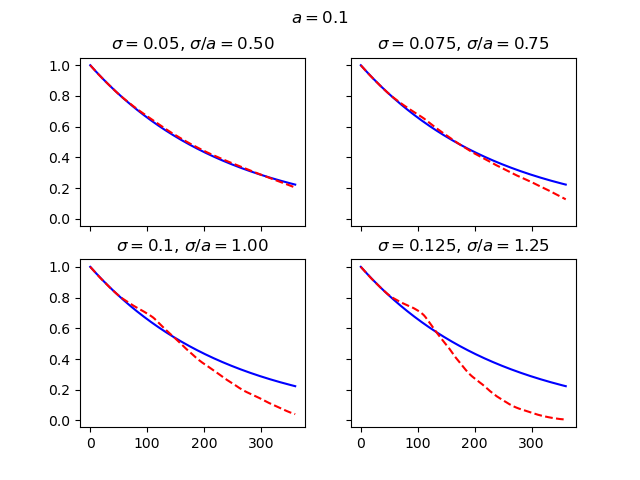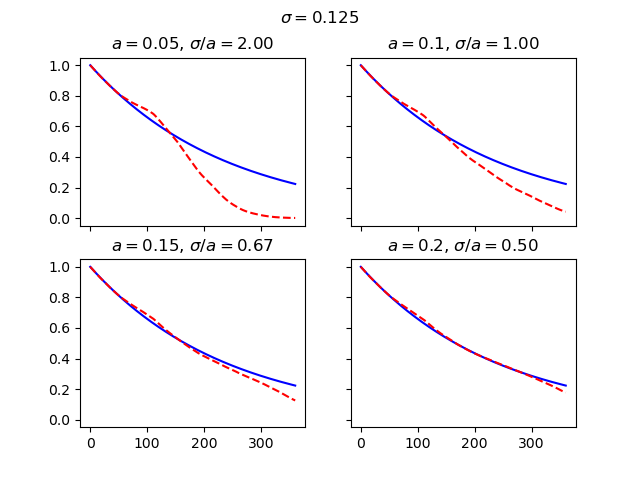# Discount factor convergence#

The convergence of the stochastic discount factors generated by the Hull-White model.

The charts below examine the convergence of the discount factors for various combinations of $$\sigma$$ and $$a$$, first by changing $$\sigma$$ and secondly by changing $$a$$. As Balaraman’s study shows, the convergence gets worse as $$\sigma/a$$ gets larger than 1, and gets better as $$\sigma/a$$ gets smaller than 1.

••import modelx as mx
import matplotlib.pyplot as plt

fig, axs = plt.subplots(2, 2, sharex=True, sharey=True)
fig.suptitle(r"$a=$" + str(HW.a))
for sigma, (h, v) in zip([0.05, 0.075, 0.1, 0.125], [(0, 0), (0, 1), (1, 0), (1, 1)]):
HW.sigma = sigma
axs[h, v].set_title(r"$\sigma=$" + str(sigma) +  r", $\sigma/a=$" + "%.2f" % (sigma/HW.a))
axs[h, v].plot(range(HW.step_size+1), [HW.mkt_zcb(i) for i in range(HW.step_size+1)], "b-")
axs[h, v].plot(range(HW.step_size+1), HW.mean_disc_factor(), "r--")

fig, axs = plt.subplots(2, 2, sharex=True, sharey=True)
fig.suptitle(r"$\sigma=$" + str(HW.sigma))
HW.sigma = 0.1
for a, (h, v) in zip([0.05, 0.1, 0.15, 0.2], [(0, 0), (0, 1), (1, 0), (1, 1)]):
HW.a = a
axs[h, v].set_title(r"$a=$" + str(a) +  r", $\sigma/a=$" + "%.2f" % (HW.sigma/HW.a))
axs[h, v].plot(range(HW.step_size+1), [HW.mkt_zcb(i) for i in range(HW.step_size+1)], "b-")
axs[h, v].plot(range(HW.step_size+1), HW.mean_disc_factor(), "r--")


Total running time of the script: ( 0 minutes 0.989 seconds)

Gallery generated by Sphinx-Gallery